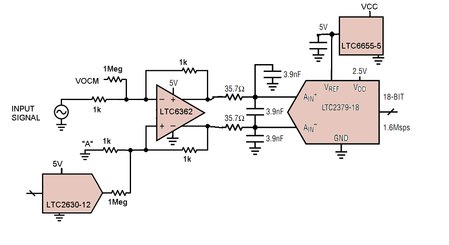Offset Adjust for a Differential Op Amp Driving an ADC

Fully differential op amps are useful components to interface a single-ended signal to a differential-input ADC. For example, the LTC6362 is a low power differential op amp that is a good choice to drive 16- and 18-bit SAR ADCs such as the LTC2379-18 family. While both of these parts already have quite good DC accuracy in terms of offset voltage, sometimes you want to further tweak the offset of the circuit. You can do this using a DAC such as the LTC2630-12, but where do you connect it into the circuit?

One obvious choice is shown in Figure 1. Node “A” is the node with respect to which the input signal is measured. So if you make small adjustments in the DC voltage at node “A”, then you are indeed adjusting the offset of the system. While this arrangement works in theory, there can be some practical problems. First, you need a quite high resolution DAC to make fine adjustments. For example, a 12-bit DAC on a 5V reference results in adjustment steps of 1.25mV, which can often be too coarse. Second, if the input signal swings symmetrical around ground (sometimes called “bipolar”), then the DAC needs to make adjustments above and below ground. That means the DAC needs a negative supply, which is not always available and in any case is not always supported by modern DACs. Finally, if the input signal changes relatively quickly, then node “A” needs to remain low impedance at these higher frequencies. Unfortunately, even buffered VOUT DACs such as LTC2630-12 have impedance on the order of 100Ohm at 100kHz, which would cause errors in the circuit operation.Figure 1. DAC Driving "A" Node Directly

Figure 2 solves all of the problems mentioned above. Rather than driving node “A” directly, the DAC connects through a high-value resistor directly into the summing node of the op amp. The resistor ratio of 1Meg/1k = 1000 divides the step size of the DAC, so that now you can make adjustments of 1.25µV using a 12-bit DAC. And it is okay for the DAC to only swing positive even if node “A” is set at ground and the input signal swings above and below ground. That’s because the common-mode level shift feature of the differential op amp ensures that the op amp input nodes are biased well above ground even when the inputs to the resistors are at ground. The second 1Meg resistor simply ensures that there is zero offset adjust when the DAC output is at mid-scale. Finally, because the DAC + 1Meg are essentially functioning like a current source rather than a voltage source, this circuit is not sensitive to output impedance of the DAC itself.Figure 2. DAC Connects To Summing Node of Op Amp Through Resistor

We built up this circuit by stringing together DC1805A and DC1074A Demo boards, featuring the LTC6362 differential op amp, LTC2379-18 SAR ADC, LTC6655-5 reference, and LTC2630-12 DAC. We verified that changing the DAC code indeed results in the expected very fine offset adjustments. We also checked that the distortion and noise of the combined circuit are not affected by connecting the DAC.

In summary, the circuit in Figure 2 is a great way to do offset adjust in a high-performance differential ADC circuit.